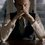# Mass is the amount of substance, what's Electromagnetic Mass?

We usually learn mass is the amount of the substance contained in it. But who might have guessed then that it was just one side of the story. That was just "mechanical mass"! There is one more type namely Electromagnetic Mass. It is all because computation tells us that momentum(due to em fields) comes out to be proportional to velocity. And what must be the coefficient of proportionality? You guessed it right. It's our deary Mass. It sometimes becomes $\frac{2{e}^{2}}{3a{c}^{2}}$ where ${e}^{2} = \frac{{q}^{2}}{4\pi\epsilon}$, $a$ is the radius, $c$ is of course speed of light in vacuum.

But it comes out to be wrong since here we have not considered the "Poincare stresses". And we have to add the two contributions.

My question however, is how you would explain this "Electromagnetic mass" physically. That will be your own opinion since I think it has not been explained. This is just a mathematical consequence. What's the physics?Note by Kartik Sharma
4 years, 8 months ago

This discussion board is a place to discuss our Daily Challenges and the math and science related to those challenges. Explanations are more than just a solution — they should explain the steps and thinking strategies that you used to obtain the solution. Comments should further the discussion of math and science.

When posting on Brilliant:

• Use the emojis to react to an explanation, whether you're congratulating a job well done , or just really confused .
• Ask specific questions about the challenge or the steps in somebody's explanation. Well-posed questions can add a lot to the discussion, but posting "I don't understand!" doesn't help anyone.
• Try to contribute something new to the discussion, whether it is an extension, generalization or other idea related to the challenge.

MarkdownAppears as
*italics* or _italics_ italics
**bold** or __bold__ bold
- bulleted- list
• bulleted
• list
1. numbered2. list
1. numbered
2. list
Note: you must add a full line of space before and after lists for them to show up correctly
paragraph 1paragraph 2

paragraph 1

paragraph 2

[example link](https://brilliant.org)example link
> This is a quote
This is a quote
    # I indented these lines
# 4 spaces, and now they show
# up as a code block.

print "hello world"
# I indented these lines
# 4 spaces, and now they show
# up as a code block.

print "hello world"
MathAppears as
Remember to wrap math in $$ ... $$ or $ ... $ to ensure proper formatting.
2 \times 3 $2 \times 3$
2^{34} $2^{34}$
a_{i-1} $a_{i-1}$
\frac{2}{3} $\frac{2}{3}$
\sqrt{2} $\sqrt{2}$
\sum_{i=1}^3 $\sum_{i=1}^3$
\sin \theta $\sin \theta$
\boxed{123} $\boxed{123}$

Sort by:

Kartik, this is a huge subject. If you want a short answer, the idea of an "electromagnetic mass" has been superseded by special relativity, it's become largely irrelevant. But if you want the long answer, wait for it. It's a very interesting and instructive subject, which should serve to show that numerous physicists in the late 19th century were well aware of the issues that ultimately led to Einstein's theory of relativity.

- 4 years, 8 months ago

Oh, thanks! I will surely want the long answer and wait. :)

- 4 years, 8 months ago

@Michael Mendrin I am not able to think of other names(my memory just went away).

- 4 years, 8 months ago